[BACK]Computer Modeling in Engineering & SciencesDOI: 10.32604/cmes.2021.014980

ARTICLE

Dynamical Analysis of Radiation and Heat Transfer on MHD Second Grade Fluid

1Department of Mathematics, University of Management and Technology, Lahore, 54770, Pakistan
2Institute for Groundwater Studies (IGS), University of the Free State, Bloemfontein, 9301, South Africa
3Department of Science & Humanities, National University of Computer and Emerging Sciences, Lahore, 54000, Pakistan
4School of Mathematics and Information Science, Henan Polytechnic University, Jiaozuo, 454000, China
*Corresponding Author: Shaowen Yao. Email: yaoshaowen@hpu.edu.cn
Received: 13 November 2020; Accepted: 08 April 2021

Abstract: Convective flow is a self-sustained flow with the effect of the temperature gradient. The density is non-uniform due to the variation of temperature. The effect of the magnetic flux plays a major role in convective flow. The process of heat transfer is accompanied by a mass transfer process; for instance, condensation, evaporation, and chemical process. Due to the applications of the heat and mass transfer combined effects in a different field, the main aim of this paper is to do a comprehensive analysis of heat and mass transfer of MHD unsteady second-grade fluid in the presence of ramped boundary conditions near a porous surface. The dynamical analysis of heat transfer is based on classical differentiation with no memory effects. The non-dimensional form of the governing equations of the model is developed. These are solved by the classical integral (Laplace) transform technique/method with the convolution theorem and closed-form solutions are attained for temperature, concentration, and velocity. The physical aspects of distinct parameters are discussed via graph to see the influence on the fluid concentration, velocity, and temperature. Our results suggest that the velocity profile decrease by increasing the Prandtl number. The existence of a Prandtl number may reflect the control of the thickness of momentum and enlargement of thermal conductivity. Furthermore, to validate our results, some results are recovered from the literature.

Keywords: Heat transfer; magnetic effect; ramped conditions; porous medium; Laplace transform; thermal radiation

1  Introduction

In the literature, different theories are made to see the phenomenon of heat transfer analysis. Radiation, convection, and conduction are three modes of heat transfer. Convection can be defined as heat transfer by the substance motion which may be air or water. It plays a central role in creating the weather clause on the plant. Convection consists of forced and natural convection. It happens when the medium divides the heat energy and is on its move. Heat is disbursed when a fan pushes air, and sometimes heat advection is referred to the forced convection. Heat is animated to distress by means of itself hotness becomes the cause of natural convection by means of shifting source heat. Heat and mass are related to each other as heat transfer rate depends on mass transfer and mass transfer further depends upon concentration difference. Free convective flow is a self-reliant flow with the effect of heat transfer. In different industrial problems, the fluid flow phenomenon of free convection has achieved much consideration in recent years. The atmospheric, oceanic circulation, and emergency cooling system of advanced nuclear reactors is the most important application of free convection. The effect of heat transfer in non-Newtonian fluids plays a major role applying engineering such as drag reduction, the emergency cooling system of nuclear reactors, and thermal welding. In literature, different theories are made to see the phenomenon of heat transfer analysis. The convection heat transform between two heated cubes was discussed by Mousazadeh et al. . Sajad et al.  investigated the heat transfer and magnetic effects on hybrid nanofluid. Iftikhar et al.  analyzed the influence of heat and mass transform with the existence of a magnetic field in the rate type fluid model. Convective flow is a self-reliant flow with the effect of the heat transfer. The influence of magnetic flux plays a significant role in convective flow. In the literature, different theories are made to see the phenomenon of heat transfer analysis. Radiation, convection and conduction are three modes of heat transfer. Convection can be defined as heat transfer by the substance motion which may be air or water. It plays a central role in creating the weather clause on the plant.

Although the research on the Second grade model can be continued, we include here some related studies based on heat transfer, fractional models of fluids, magnetized fluids, and few others therein . Motivating by above discussion, this manuscript aims to explore the unique and exact solution of MHD Second grade model with simultaneous use of ramped heating with ramped velocity near a porous surface. Laplace integral transformation is used to obtain the unique solution of velocity, temperature, and concentration under impact of ramped heating. In Section 2, the dimensionless governing equations are developed. In Section 3, Laplace integral transform is used to find the exact solution of the temperature, velocity, and concentration field. In Section 4, some existing results are recovered as the limiting cases to justify our results. In Section 5, the effect of physical parameters is analyzed graphically. The concluding observation is listed at the end.

2  Development of Governing Equations Based on Problem Statement

Consider the MHD Second grade fluid flow in a porous medium over an limitless vertical plate. Initially, the fluid is at rest at t = 0. After t < t0, the T(TTwtt0) is the plate temperature with velocity w0tt0. At t > t0, it give constant velocity w0 with temperature T. The fluid flow is one dimensional. The flow direction of radiative flux is negligible. Finally in energy equation, the dispersion term is neglected. The geometrical presentation of considered model is provided in Fig. 1. Under some these presumptions, the governing equation for MHD Second grade fluid with appropriate conditions are given below :

w(ζ,t)t=υ(1+α1μt)2w(ζ,t)ζ2+gβT(TT)+gβC(CC)σ0M02ρw(ζ,t)υϕk0(1+α1μt)w(ζ,t),(1)

Tt=kρCp(1+16σ1T33kK1)2Tζ2, (2)

Ct=Dm2Cζ2Dm(CC), (3)

with appropriate conditions

w(ζ,0)=0,T(ζ,0)=T,C(ζ,0)=C,w(ζ,0)t=0,ζ0, (4)

C(0,t)=Cw,w(0,t)=f1(t),T(0,t)=f2(t), (5)

where

f1(t)={w0tt0,0<tt0;w0,t>t0} and f2(t)={T+(TwT)tt0,0<tt0;T(0,t)=Tw,t>t0},

w(ζ,t)0,T(ζ,t),C(ζ,t) as ζ. (6)

Consider the different dimensionless quantities

ψ=w03υζ,τ=w02υt,V=ww03,θ=TTTwT,ϕ=CCCwC,Gr=gβTt0(TT)w03,

Gm=gβCt0(CC)w03,M=σ0M02υρw02,Nr=16σ1T33kK1,Pr=υCpk,Preff=Pr1+Nr, (7)

1K=υ2ϕk0w02,α=α1ρw02μ2,Sc=υDm,r=M+1K,s=αK,λ=υ2w02.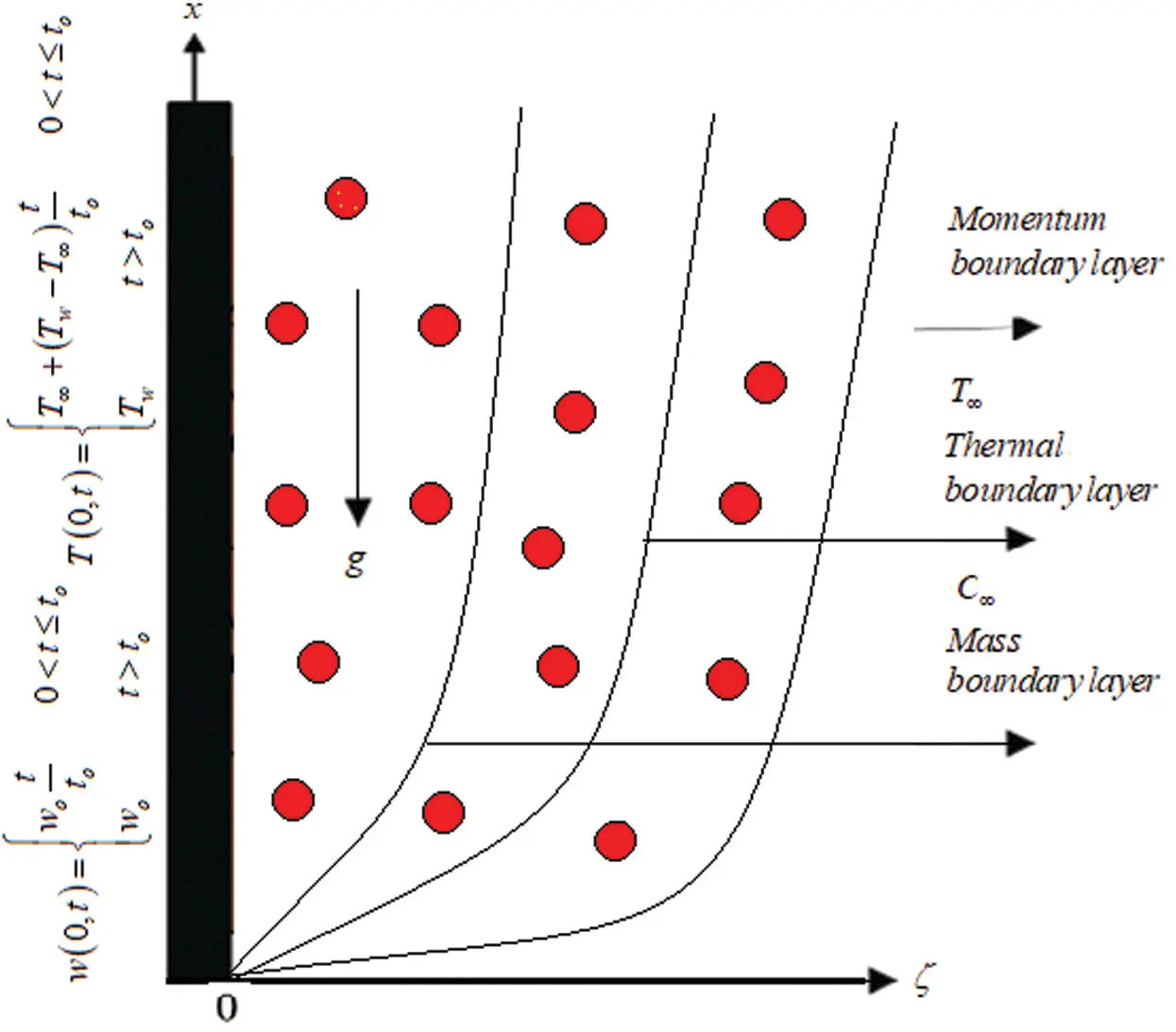Figure 1: Geometrical presentation for second grade model

After simplification, we have the set of dimensionless governing equations and their corresponding conditions are:

V(ψ,τ)τ=2V(ψ,τ)ψ2+Grθ(ψ,τ)+Gmϕ(ψ,τ)rV(ψ,τ)sV(ψ,τ)τ+α3V(ψ,τ)τψ2, (8)

θ(ψ,τ)τ=1Preff2θ(ψ,τ)ψ2, (9)

ϕ(ψ,τ)τ=1Sc2ϕ(ψ,τ)ψ2λScϕ(ψ,τ), (10)

V(ψ,0)=θ(ψ,0)=ϕ(ψ,0)=0, (11)

V(0,τ)=θ(0,τ)=f(τ) where f(τ)={τ,0<τ11,τ>1},ϕ(0,τ)=1, (12)

V(,τ)0,θ(,τ)0,ϕ(,τ)0,ψ. (13)

3  Exact Solution of the Problem

3.1 Solution of Heat Profile

Applying Laplace transformation to get the solution of Eq. (9) using Eqs. (11)(13), we have

2θc(ψ,m)ψ2mPreffθc(ψ,m)=0. (14)

The solution of above Eq. (14) is

θc(ψ,m)=c1eψmPreff+c2eψmPreff, (15)

using appropriate conditions are used to find the value of constant,

θc(ψ,m)=(1emm2)eψmPreff, (16)

θc(ψ,m)=(eψmPreffm2)em(eψmPreffm2)=θrc(ψ,m)emθrc(ψ,m). (17)

The inverse Laplace transformation of Eq. (17) is

θ(ψ,τ)=θr(ψ,τ)θr(ψ,τ0)H(τ0), (18)

θr(ψ,τ)=(Preff2ψ2+τ)erfc(Preff4τψ)(Preffτπψ)ePreffψ24τ, (19)

where H(τ0) represent a standard Heaviside function with τ0 = τ − 1.

3.1.1 Nusselt Number

To estimate the rate of heat transfer can be obtained as

Nu=θ(ψ,τ)ψ|ψ=0. (20)

3.2 Solution of Mass Profile

Applying the Laplace transformation to Eq. (10) with suitable initial condition on concentration

2ϕc(ψ,m)ψ2(mSc+λ)ϕc(ψ,m)=0. (21)

The required solution of second order differential Eq. (21) with help of (11)(13) on concentration is given by

ϕc(ψ,p)=1meψmSc+λ. (22)

To obtain the exact solution, we take the inverse Laplace transformation, we have

ϕ(ψ,τ)=12(eψScλScerfc(ψSc2τλScτ))+12(eψScλScerfc(ψSc2τ+λScτ)), (23)

using from literature

L1{eψcp+bpa}=eaτ2(eψca+berfc(ψc2τ(a+b)τ))

+eaτ2(eψca+berfc(ψc2τ+(a+b)τ)). (24)

3.3 Solution of Velocity Profile

The solution of Eq. (8) by using Laplace transform, we have

(r+(1+s)m)Vc(ψ,m)=(1+αm)2Vc(ψ,m)ψ2+Grθc(ψ,m)+Gmϕc(ψ,m), (25)

by using the Eqs. (16) and (22) for the values θc(ψ,m) and ϕc(ψ,m), the solution of Eq. (25) can be written as

Vc(ψ,m)=c1eψr+(1+r)m1+αm+c2eψr+(1+s)m1+αm(Gr(1em)Preffm(r+(1+s)m1+αm))eψPreffm(1+αm)m2 (GmScm+λ(r+(1+s)m1+αm))eψScm+λ(1+αm)m,(26)

Vc(ψ,m)=(1emm2)eψr+(1+s)m1+αm+(1emm2)(GrαPreff)×(eψr+(1+s)m1+αmeψPreffm(m+n1)2(n2)2)+(GmmαSc)×(eψr+(1+s)m1+αmeψScm+λ(m+n3)2(n4)2),(27)

where

n1=Preff(1+s)2αPreff,n2=(Preff(1+s)2αPreff)2+rαPreff,

n3=Sc+αλ(1+s)2αSc,n4=(Sc+αλ(1+s)2αSc)2λrαSc. (28)

Eq. (27) can be written as in the following form:

Vc(ψ,m)=Sc(ψ,m)emSc(ψ,m)+Rc(ψ,m), (29)

where

Sc(ψ,m)=(eψr+(1+s)m1+αmm2)+(GrαPreffm2)(eψr+(1+s)m1+αmeψPreffm(m+n1)2(n2)2), (30)

Rc(ψ,m)=(GmmαSc)(eψr+(1+s)m1+αmeψScm+λ(m+n3)2(n4)2), (31)

Further, Sc(ψ,m) can be written as

Sc(ψ,m)=S1c(ψ,m)+[S1c(ψ,m)θrc(ψ,m)]S2c(m),Rc(ψ,m)=[R1c(ψ,p)ϕc(ψ,m)]R2c(m), (32)

where

S1c(ψ,m)=(eψr+(1+s)m1+αmm2),S2c(m)=(GrαPreff)(1(m+n1)2(n2)2),

R1c(ψ,m)=(eψr+(1+s)m1+αmm) and R2c(m)=(GmαSc)(1(m+n3)2(n4)2). (33)

Applying inverse Laplace transformation with convolution product on Eq. (32), we get the required solution

S1(ψ,τ)=1+sα00τe(w(1+s)+z)αerfc(ψ2z)I0(2α(1+srα)wz)dzdw

+rα00ν0τe(w(1+r)+m)αerfc(ψ2z)I0(2α(1+srα)qw)dzdqdw, (34)

S2(τ)=Gren1τPreff[sinh(n2τ)αn2], (35)

R1(ψ,τ)=eψe1ψe22π00τ1τe(e3τ+ψ24v+e1v)I1(2e2vτ)dτdv, (36)

R2(τ)=Gmen3τSc[sinh(n4τ)αn4]. (37)

Substituting all the above functions, the equation of velocity field becomes in the form

V(ψ,τ)=S(ψ,τ)S(ψ,τ0)H(τ0)+R(ψ,τ). (38)

3.3.1 Skin Friction

The following relation used to calculate the skin friction as

Cf=V(ψ,τ)ψ|ψ=0. (39)

4  Limiting Cases

We recover the same result for velocity field in the absence of mass Grashof number Gm = 0 obtained by Anwar et al. . Also when Nr = 0 and Gm = 0, we get the same expression for velocity as discussed by Samiulhaq et al. .

5  Graphical Discussion

This part is devoted for physical interpretation of heat transfer is executed on the motion of Second-grade fluid near a porous surface. The impact of thermal radiation, magnetic field, and ramped temperature conditions are also analyzed via Laplace integral transform to obtain a unique and exact solution. The graphical representations are depicted for showing the impact of physical parameters such as Pr, Nr, Sc, Gm, Gr, K and M on energy, concentration and velocity profile. Fig. 2 is plotted for the impact of Pr on energy profile. It is seen that thermal layer and temperature decreases by large value of Pr. As Pr increase, the temperature profile reduce more rapidly. Physically, for less value of Pr heat conductivity enhances. Fig. 3 shows the impact of heat radiation Nr. By enhancing the value of Nr, the required energy enhance. The domination of Nr, enhanced due to transportation of energy.Figure 2: Temprature for altered values of Pr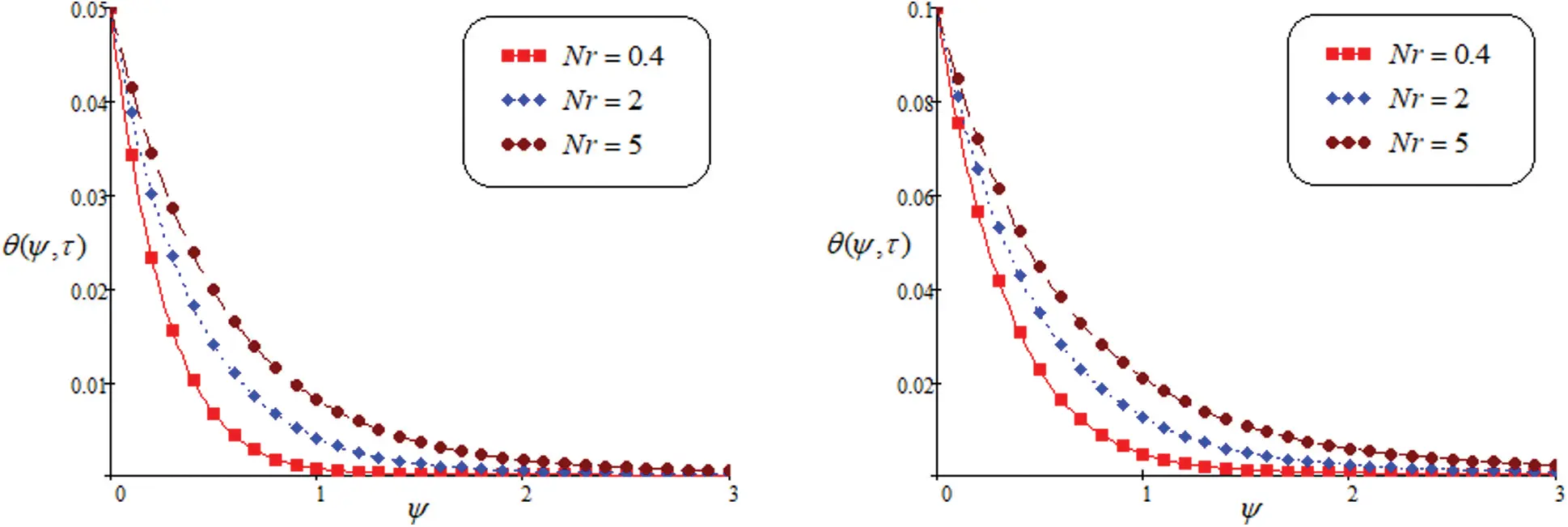Figure 3: Temprature for altered values of Nr

In Figs. 4 and 5, we discussed the behavior of λ and Sc on concentration profile. It is noted that the greater value of λ, the concentration is increasing while in case of Sc, the concentration reduce as enhance the value of Sc. Contribution of concentration component on the fluid motion is important and it cannot be ignored.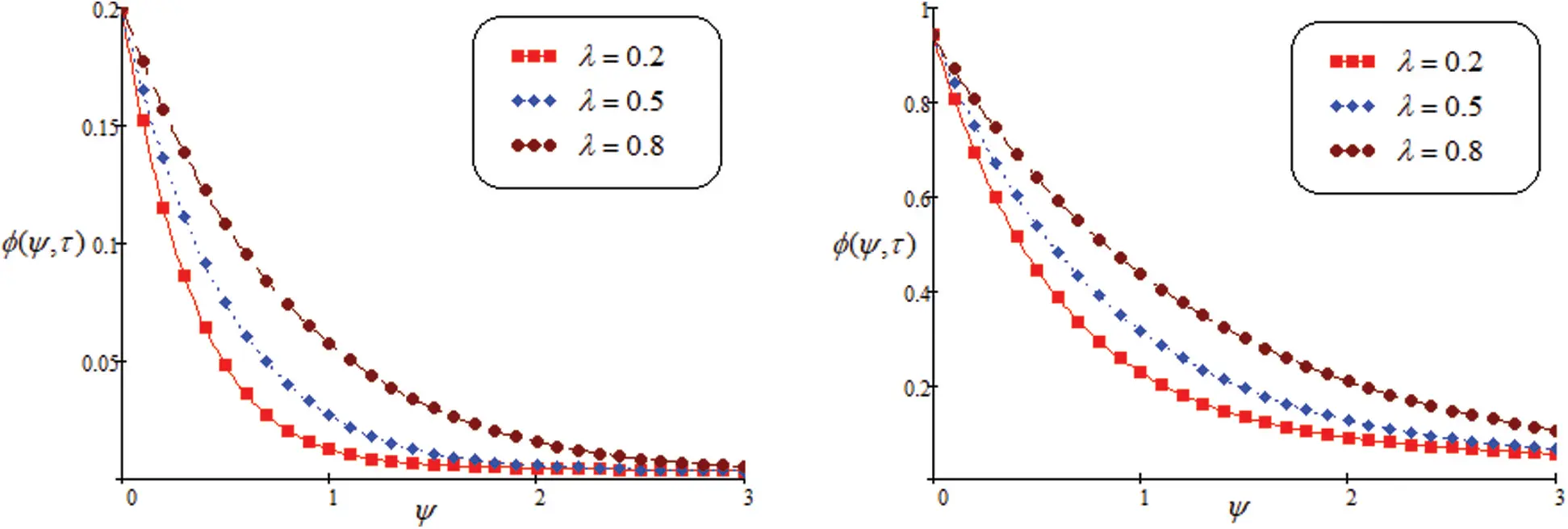Figure 4: Concentration for altered values of λ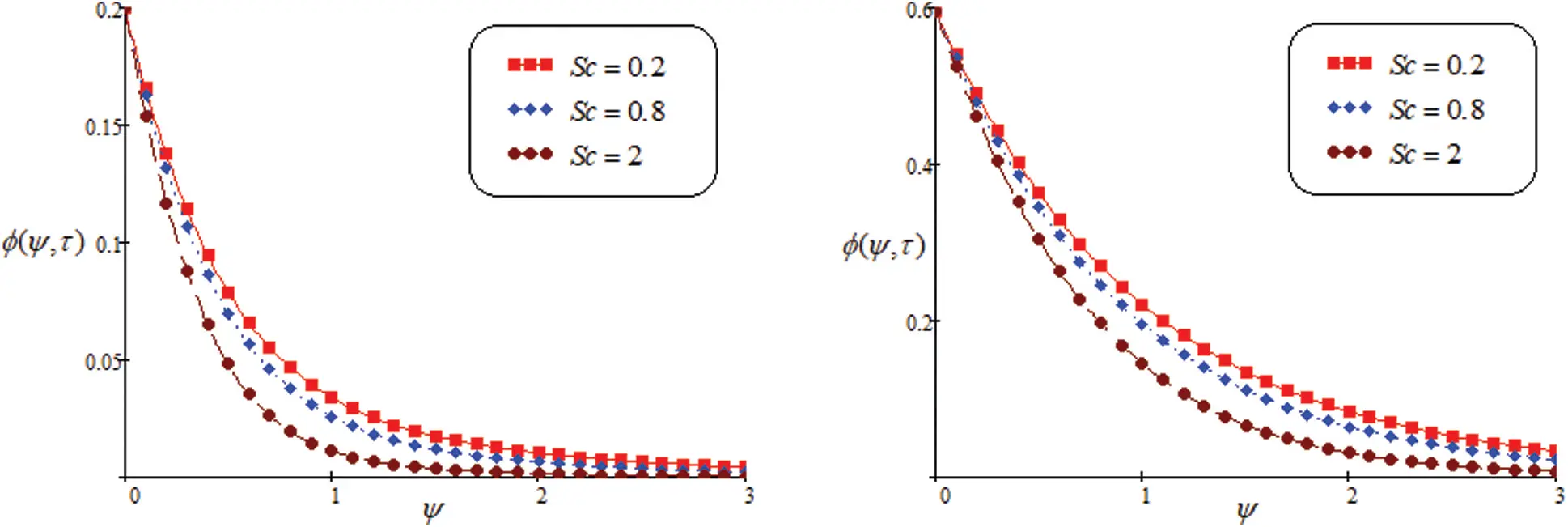Figure 5: Concentration for altered values of Sc

Fig. 6 shows the influence of Gm. It is the ratio of mass force to viscous force, which causes free convection. It is noted that the fluid velocity is increasing, if we increase the value of Gm. Fig. 7 shows that the impact on Gr for velocity field. The curve behavior of Gr is same as Gm. It is noted that the velocity field enhance by increasing in Gr. Physically, when the Gr is increased, then fluid flow increase due to the thermal effects.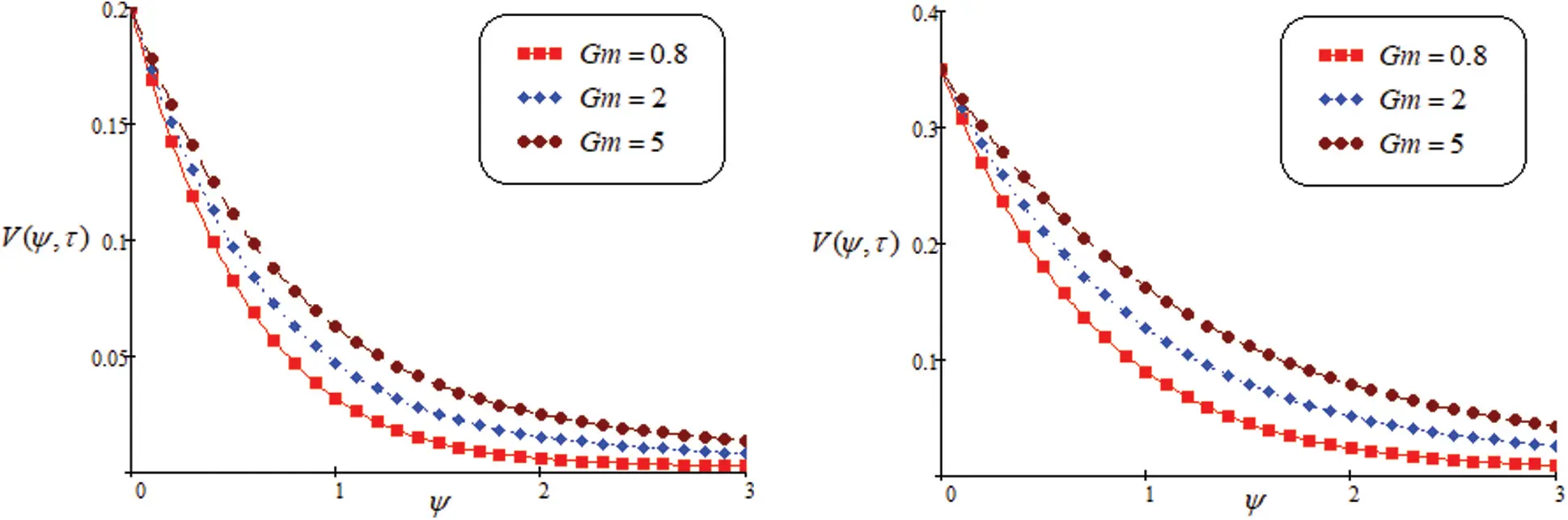Figure 6: Velocity for altered values of Gm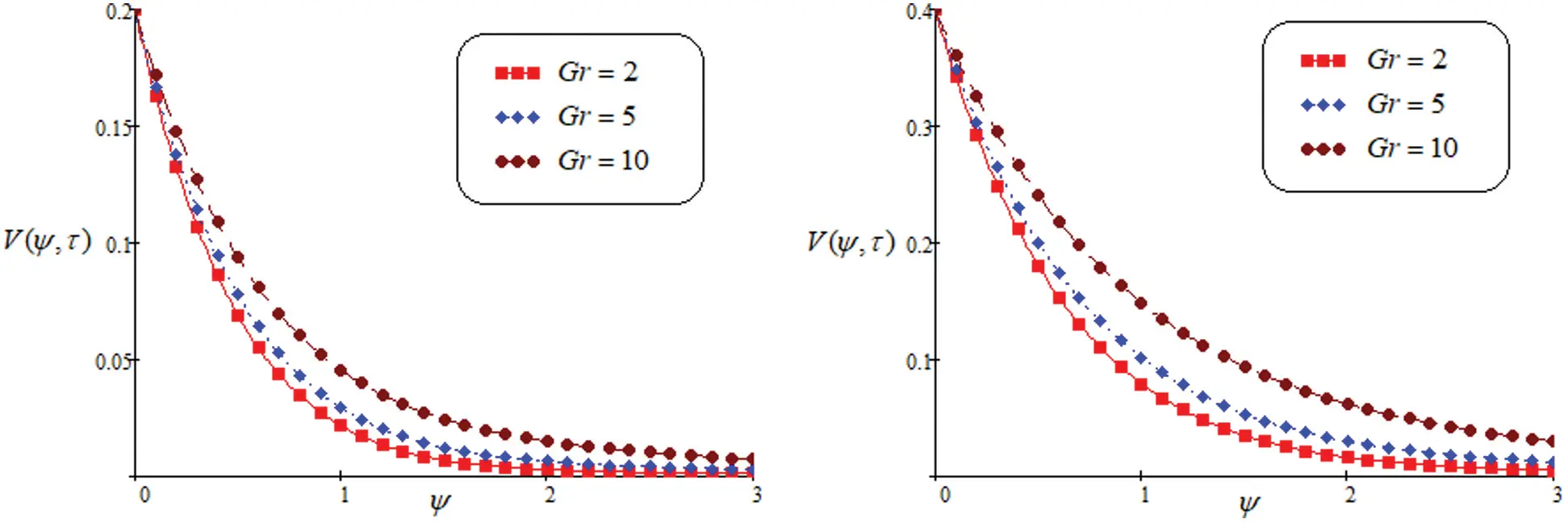Figure 7: Velocity for altered values of Gr

Fig. 8 shows the effect of permeability on velocity curve. The fluid velocity enhance as escalate the value of K. Fig. 9 discusses the domination of M on the velocity curve. These graphical representation indicates that an increase in M, the velocity reduce due to Lorentz force. It behave as a drag force. By increasing the parameter of magnetic field, the Lorentz force also increase. Fluid flow on the boundary layer is slow down due to this force. Fig. 10 discusses the behavior of Pr. Specific heat and conductivity are depend on Pr. The thickness of momentum and boundary layer is controled by prandtl number. It seen from the graph, decreasing the velocity, observed by increase the value of Pr. The lower prandtl number enhance the thermal conductivity and increase the boundary layer. Fig. 11 shows the impact of Sc on fluid profile. It notices that the value of Sc enhance, the velocity of fluid flow decreased.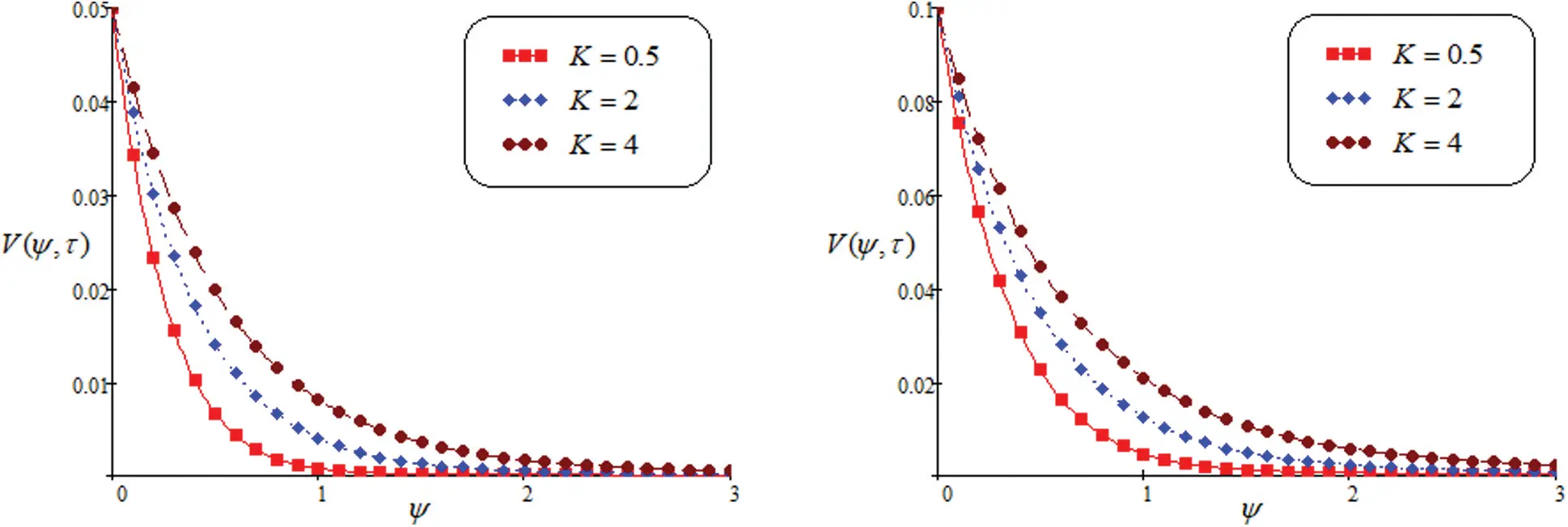Figure 8: Velocity for altered values of K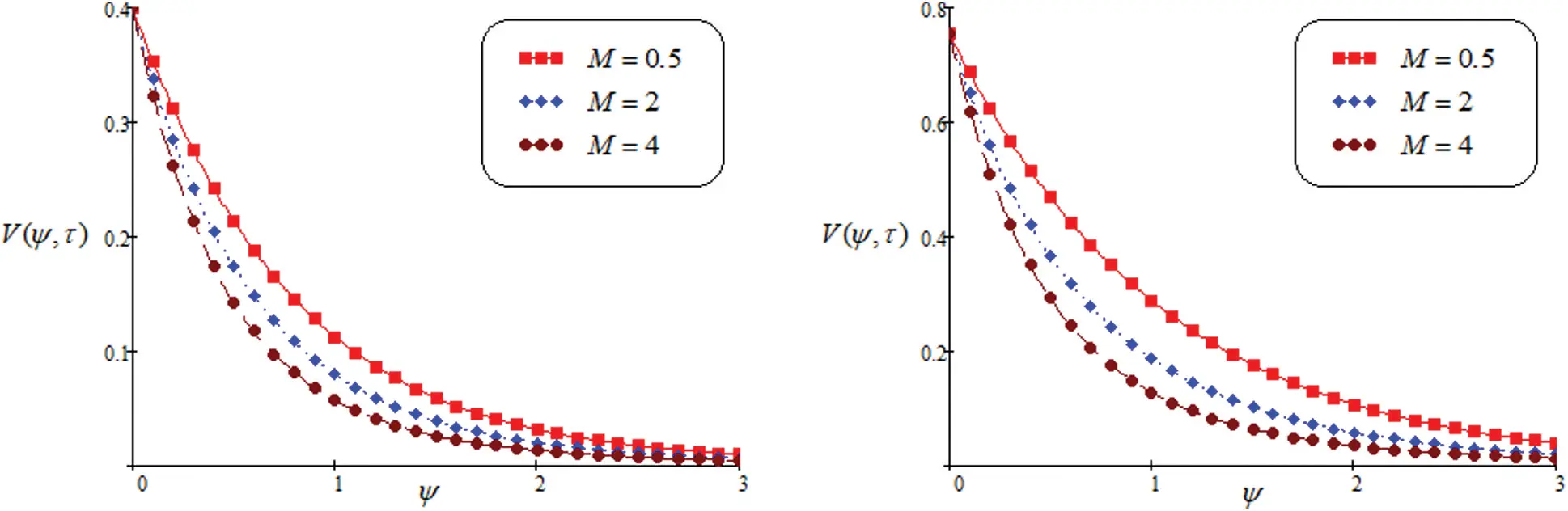Figure 9: Velocity for altered values of MFigure 10: Velocity for altered values of Pr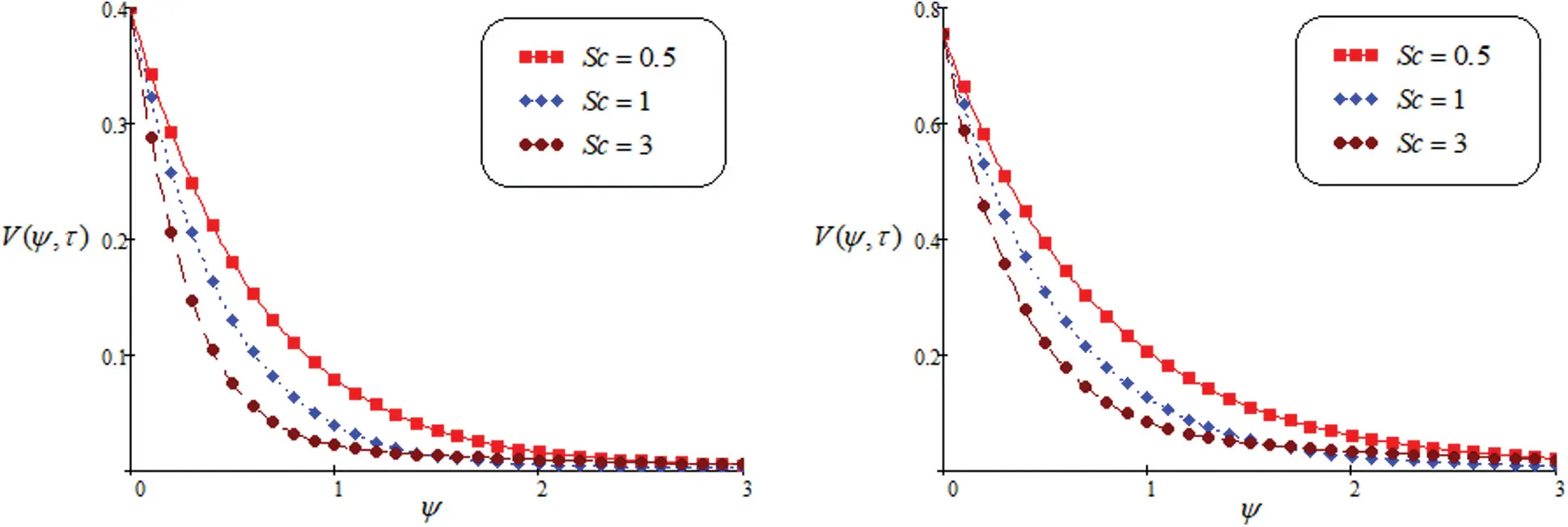Figure 11: Velocity for altered values of Sc

6  Concluding Observation

This part is devoted for dynamical analysis of heat transfer on MHD Second-grade fluid near a porous surface. The impact of ramped temperature condition and magnetic effect are analyzed via Laplace integral transform to obtain a unique and exact solution of velocity profile. Some significant remarks and concluding results are given below:

•   It is noted that the decay in temperature for increasing value of Pr while temperature is increasing as increase in Nr.

•   The decay in concentration for growing the values of Sc.

•   The fluid velocity decreases when the value of M increased.

•   Enhance the values of Gr, Gm, the required fluid velocity is increasing.

•   Enhance the values of K, the required fluid velocity is increasing.

•   In case of large value of Sc and magnetic field M the fluid velocity is decelerated.

•   Contribution of concentration component of fluid velocity on the fluid motion is important and it cannot be ignored.

Acknowledgement: The authors are highly thankful and grateful for generous support and facilities of this research work.

Funding Statement: Self supported by the authors.

Conflicts of Interest: The authors declare that they have no conflicts of interest to report regarding the present study.

## References

1. Mousazadeh, S. M., Shahmardan, M. M., Tavangar, T., Hosseinzadeh, K., & Ganji, D. D. (2018). Numerical investigation on convective heat transfer over two heated wall-mounted cubes in tandem and staggered arrangement. Theoretical and Applied Mechanics Letters, 8(3), 171-183. [Google Scholar] [CrossRef]
2. Sajad, S., Nori, A., Hosseinzadeh, K., & Ganji, D. D. (2020). Hydrothermal analysis of MHD squeezing mixture fluid suspended by hybrid nanoparticles between two parallel plates. Case Studies in Thermal Engineering, 21(5), 100650. [Google Scholar] [CrossRef]
3. Iftikhar, N., Husnine, S. M., & Riaz, M. B. (2019). Heat and mass transfer in MHD maxwell fluid over an infinite vertical plate. Journal of Prime Research in Mathematics, 15, 63-80. [Google Scholar]
4. Qi, H., & Jin, H. (2006). Unsteady rotating flows of a viscoelastic fluid with the fractional maxwell model between coaxial cylinders. Acta Mechanica Sinica, 22(4), 301-305. [Google Scholar] [CrossRef]
5. Hosseinzadeh, K., Mogharrebi, A. R., Asadi, A., Paikar, M., & Ganji, D. D. (2020). Effect of fin and hybrid nano-particles on solid process in hexagonal triplex latent heat thermal energy storage system. Journal of Molecular Liquids, 300(2), 112347. [Google Scholar] [CrossRef]
6. Gholinia, M., Gholinia, S., Hosseinzadeh, K., & Ganji, D. D. (2018). Investigation on ethylene glycol nano fluid flow over a vertical permeable circular cylinder under effect of magnetic field. Results in Physics, 9, 1525-1533. [Google Scholar] [CrossRef]
7. Rahimi, J., Ganji, D. D., Khaki, M., & Hosseinzadeh, K. (2017). Solution of the boundary layer flow of an Eyring–Powell non-Newtonian fluid over a linear stretching sheet by collocation method. Alexandria Engineering Journal, 56(4), 621-627. [Google Scholar] [CrossRef]
8. Hosseinzadeh, K., Mogharrebi, A. R., Asadi, A., Mogharrebi, A. R., & Ganji, D. D. (2020). Effect of internal fins along with hybrid nanoparticles on solid process in star shape triplex latent heat thermal energy storage system by numerical simulation. Renewable Energy, 154(2), 497-507. [Google Scholar] [CrossRef]
9. Sobral, D. C. (2003). A new proposal to guide velocity and inclination in the ramp protocol for the Treadmill Ergometer. Arquivos Brasileiros de Cardiologia, 81, 48-53. [Google Scholar] [CrossRef]
10. Bruce, R. A. (1956). Evaluation of functional capacity and exercise tolerance of cardiac patients. Modern Concepts of Cardiovascular Disease, 25, 321-326. [Google Scholar]
11. Myers, J., & Bellin, D. (2000). Ramp exercise protocol for clinical and cardiopulmonary exercise testing. Sports Medicine, 30(1), 23-29. [Google Scholar] [CrossRef]
12. Kundu, B. (2016). Exact analysis for propagation of heat in a biological tissue subject to different surface conditions for therapeutic applications. Applied Mathematics and Computation, 285(2), 204-216. [Google Scholar] [CrossRef]
13. Riaz, M. B., Atangana, A., Saeed, S. T. (2020). MHD free convection flow over a vertical plate with ramped wall temperature and chemical reaction in view of non-singular kernel. Fractional Order Analysis: Theory, Methods and Applications, 253–282. DOI 10.1002/9781119654223.ch10. [CrossRef]
14. Abdeljawad, T., Riaz, M. B., Saeed, S. T., & Iftikhar, N. (2021). MHD maxwell fluid with heat transfer analysis under ramp velocity and ramp temperature subject to non-integer differentiable operators. Computer Modeling in Engineering & Sciences, 126(2), 821-841. [Google Scholar] [CrossRef]
15. Iftikhar, N., Saeed, S. T., Riaz, M. B. (2021). Fractional study on heat and mass transfer of MHD Oldroyd-B fluid with ramped velocity and temperature. Computational Methods for Differential Equations, 1–28. DOI 10.22034/cmde.2021.39703.1739. [CrossRef]
16. Saeed, S. T., Khan, I., Riaz, M. B., & Husnine, S. M. (2020). Study of heat transfer under the impact of thermal radiation, ramped velocity, and ramped temperature on the MHD Oldroyd-B fluid subject to noninteger differentiable operators. Journal of Mathematics, 2020(2), 1-14. [Google Scholar] [CrossRef]
17. Ahmed, N., & Dutta, M. (2013). Transient mass transfer flow past an impulsively started infinite vertical plate with ramped plate velocity and ramped temperature. International Journal of Physical Sciences, 8, 254-263. [Google Scholar] [CrossRef]
18. Schetz, J. A. (1963). On the approximate solution of viscous-flow problems. Journal of Applied Mechanics, 30(2), 263-268. [Google Scholar] [CrossRef]
19. Hayday, A. A., Bowlus, D. A., & McGraw, R. A. (1967). Free convection from a vertical flat plate with step discontinuities in surface temperature. Journal of Applied Mechanics, 89, 244-249. [Google Scholar] [CrossRef]
20. Das, S., Jana, M., & Jana, R. N. (2011). Radiation effect on natural convection near a vertical plate embedded in porous medium with ramped wall temperature. Open Journal of Fluid Dynamics, 1(1), 1-11. [Google Scholar] [CrossRef]
21. Seth, G. S., & Ansari, M. S. (2010). MHD natural convection flow past an impulsively moving vertical plate with ramped wall temperature in the presence of thermal diffusion with heat absorption. International Journal of Applied Mechanics and Engineering, 15, 199-215. [Google Scholar]
22. Maiti, D. K., & Mandal, H. (2019). Unsteady slip flow past an infinite vertical plate with ramped plate temperature and concentration in the presence of thermal radiation and buoyancy. Journal of Engineering Thermophysics, 28(3), 431-452. [Google Scholar] [CrossRef]
23. Seth, G. S., Hussain, S. M., & Sarkar, S. (2015). Hydromagnetic natural convection flow with heat and mass transfer of a chemically reacting and heat absorbing fluid past an accelerated moving vertical plate with ramped temperature and ramped surface concentration through a porous medium. Journal of the Egyptian Mathematical Society, 23(1), 197-207. [Google Scholar] [CrossRef]
24. Seth, G. S., & Sarkar, S. (2015). Hydromagnetic natural convection flow with induced magnetic field and nth order chemical reaction of a heat absorbing fluid past an impulsively moving vertical plate with ramped temperature. Bulgarian Chemical Communications, 47(1), 66-79. [Google Scholar]
25. Tiwana, M. H., Mann, A. B., Rizwan, M., Maqbool, K., Javeed, S., & et, al. (2019). Unsteady magnetohydrodynamic convective fluid flow of Oldroyd-B model considering ramped wall temperature and ramped wall velocity. Mathematics, 7(8), 676. [Google Scholar] [CrossRef]
26. Anwar, T., Khan, I., Kumam, P., & Watthayu, W. (2019). Impacts of thermal radiation and heat consumption/generation on unsteady MHD convection flow of an Oldroyd-B fluid with ramped velocity and temperature in a generalized darcy medium. Mathematics, 8(1), 130. [Google Scholar] [CrossRef]
27. Anwar, T., Kumam, P., & Watthayu, W. (2020). Influence of ramped wall temperature and ramped wall velocity on unsteady magnetohydrodynamic convective maxwell fluid flow. Symmetry, 3(3), 392. [Google Scholar] [CrossRef]
28. Anwar, T., Kumam, P., Asifa, Khan., & I., Thounthong, P. (2020). An exact analysis of radiative heat transfer and unsteady MHD convective flow of a second grade fluid with ramped wall motion and temperature. Heat Transfer, 50(1), 196-219. [Google Scholar] [CrossRef]
29. Izadi, M., Sheremet, M. A., Mehryan, S. A. M., Pop, I., & Öztop, H. F. (2020). MHD thermo gravitational convection and thermal radiation of a micropolar nanoliquid in a porous chamber. International Communications in Heat and Mass Transfer, 110, 104409. [Google Scholar] [CrossRef]
30. Das, S., & Jana, R. N. (2015). Natural convective magneto-nanofluid flow and radiative heat transfer past a moving vertical plate. Alexandria Engineering Journal, 54(1), 55-64. [Google Scholar] [CrossRef]
31. Sheikholeslami, M., Ganji, D. D., Javed, M. Y., & Ellahi, R. (2015). Effect of thermal radiation on magnetohydrodynamics nanofluid flow and heat transfer by means of two phase model. Journal of Magnetism and Magnetic Materials, 374(1), 36-43. [Google Scholar] [CrossRef]
32. Gangadhar, K., Vijayakumar, D., Chamkha, A. J., Kannan, T., & Sakthivel, G. (2020). Effects of Newtonian heating and thermal radiation on micropolar ferrofluid flow past a stretching surface: Spectral quasi-linearization method. Heat Transfer, 49(2), 838-857. [Google Scholar] [CrossRef]
33. Seth, G. S., Bhattacharyya, A., Kumar, R., & Mishra, M. K. (2019). Modeling and numerical simulation of hydromagnetic natural convection casson fluid flow with nth-order chemical reaction and newtonian heating in porous medium. Journal of Porous Media, 22(9), 1141-1157. [Google Scholar] [CrossRef]
34. Samiulhaq, ., Ahmad, S., Vieru, D., Khan, I., & Shafie, S. (2014). Unsteady magnetohydrodynamic free convection flow of a second grade fluid in a porous medium with ramped wall temperature. PLoS One, 9(5), e88766. [Google Scholar] [CrossRef]
35. Siddique, I., Tlili, I., Bukhari, M., Mahsud, Y. (2019). Heat transfer analysis in convective flows of fractional second grade fluids with Caputo–Fabrizio and Atangana–Baleanu derivative subject to Newtonion heating. Mechanics of time-dependent materials. Berlin, Germany: Springer.
36. Riaz, M. B., Saeed, S. T., & Baleanu, D. (2020). Role of magnetic field on the dynamical analysis of second grade fluid: An optimal solution subject to non-integer differentiable operators. Journal of Applied and Computational Mechanics, 7(1), 54-68. [Google Scholar] [CrossRef]
37. Riaz, M. B., Siddiqui, I., Saeed, S. T., & Atangana, A. (2020). MHD Oldroyd-B fluid with slip condition in view of local and nonlocal kernels. Journal of Applied and Computational Mechanics, 7(1), 116-127. [Google Scholar] [CrossRef]
38. Tassaddiq, A. (2019). MHD flow of a fractional second grade fluid over an inclined heated plate. Chaos Solitons & Fractals, 123(5), 341-346. [Google Scholar] [CrossRef]
39. Saeed, S. T., Riaz, M. B., Baleanu, D., & Abro, K. A. (2020). A mathematical study of natural convection flow through a channel with non-singular kernels: An application to transport phenomena. Alexandria Engineering of Journal, 59(4), 2269-2281. [Google Scholar] [CrossRef]
40. Sene, N. (2020). Second-grade fluid model with Caputo–Liouville generalized fractional derivative. Chaos Solitons & Fractals, 133(3), 109631. [Google Scholar] [CrossRef]This work is licensed under a Creative Commons Attribution 4.0 International License, which permits unrestricted use, distribution, and reproduction in any medium, provided the original work is properly cited.